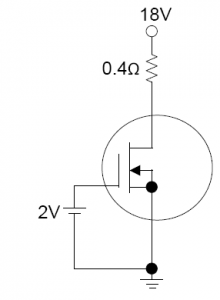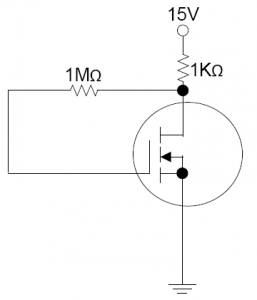# 17CS32 Analog and Digital Electronics Module 1

[wptelegram-join-channel]

## 17CS32 Analog and Digital Electronics Module 1

The important question from module 1 of Analog and Digital Electronics along with answers. Download module 1 question and answers 17CS32 Analog and Digital Electronics CBCS Notes.

### Module 1  Important questions of Analog and Digital Electronics

Notes contain answers to following questions,

1. Explain the construction and principle of operation of N-Channel and P-Channel Junction Field Effect Transistor (JFET).

2. Explain the Characteristics of N-Channel and P-Channel Junction Field Effect Transistor.

3. Explain the construction and principle of operation of N-Channel and P-Channel Depletion Metal Oxide Semiconductor Field Effect Transistor (DE-MOSFET).

4. Explain the characteristics of N-channel DE-MOSFET.

5. Explain the construction and principle of operation of N-Channel and P-Channel Enhancement Metal Oxide Semiconductor Field Effect Transistor (E-MOSFET).

6. Explain the characteristics of N-channel E-MOSFET.

7. Mention the difference between JFET and MOSFET.

8. Discuss the handling of MOSFET.

9. Discuss the application of Field Effect Transistors (FET).

10. Explain the working of CMOS inverter device.

11. The figure below shows a biasing configuration using DE-MOSFET. Given that the saturation drain current is 8mA and the pinch-off voltage is -2V, determine the value of gate-source voltage, drain current and the drain-source voltage.12. Design a voltage-divider–bias network using a DE-MOSFET with the supply voltage VDD=16V, IDSS=10mA, and VP = -5V to have a quiescent drain current of 5mA and gate voltage of 4V. (Assume the drain resistor RD to be four times the source resistor RS).

13. The figure below shows a circuit using E-MOSFET. Given that the threshold voltage for the MOSFET is 2V and ID (on) =6mA for VGS (on) =5V, determine the value of the operating point.14. Explain the Astable Operation of multivibrator using 555 Timer IC.

15. Explain the Monostable Operation of multivibrator using 555 Timer IC.

16. Discuss the Ideal Opamp versus practical Opamp.

17. Discuss the performance parameters of an operational amplifier.

#### 18. Explain the Peak Detector Circuit.

19. Explain the following comparators: (a) Zero crossing detector (b) Comparator with reference (c) Comparator with hysteresis (d)  Window comparator.

20. Explain the Relaxation Oscillator using an operational amplifier.

21. Explain the Active Filters.

22. Explain the non-linear amplifier.

23. Explain the current to voltage converter and the voltage to current converter.